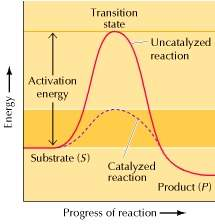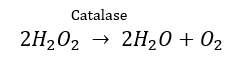Disclaimer: This is an example of a student written essay.

Any scientific information contained within this essay should not be treated as fact, this content is to be used for educational purposes only and may contain factual inaccuracies or be out of date.

# Effect of Sucrose Solution Molarity on the Rate of Catalase Enzyme Activity

 ✅ Paper Type: Free Essay ✅ Subject: Biology ✅ Wordcount: 1309 words ✅ Published: 18th May 2020

Abstract/Purpose

The purpose of this experiment was to measure the effect of sucrose solution molarity on the rate of catalase enzyme activity. Sucrose solutions of varying molarities (0.0M, 0.1M, 0.3M, and 0.5M) were introduced to a catalase enzyme, which then catalyzed the decomposition of a 1.5% hydrogen peroxide solution. The pressure created by the release of CO2 was measured in kPa using a PASCO Wireless Pressure Sensor, which was connected to a smartphone so data could be collected. The rate of enzyme activity was then calculated in kPa/sec using a graph generated by the sensor. This procedure was performed twice for each sucrose solution to calculate an average rate of enzyme activity for each. The 0.0M sucrose solution had an average rate of 0.038 kPa/sec. The 0.1M sucrose solution had an average reaction rate of 0.028 kPa/sec, the 0.3M sucrose solution had an average reaction rate of 0.071 kPa/sec, and the 0.5M sucrose solution had an average reaction rate of 0.078 kPa/sec. Based on these results, it can be concluded that in general, the higher the molarity of the sucrose solution, the higher the rate of catalase enzyme activity.

Introduction/Hypothesis

The essential question of this experiment was to determine how different molarities of sucrose solutions affect the rate of catalase enzyme activity. The rate of enzyme activity is important to living things because they speed up chemical reactions and break down substrates. Without enzymes, reactions essential to life would take place at such a slow rate that life would be unsustainable (Cooper, 2000).

If you need assistance with writing your essay, our professional essay writing service is here to help!

Enzymes are macromolecules that act as a catalyst in reactions. They bind to and break apart substrates, which is the reactant in the reaction that the enzyme is catalyzing. Enzymes catalyze reactions by reducing the activation energy of the reaction, which is the amount of energy a reaction needs to start (Reese, et al., 2011). This can be seen in the diagram below:(Cooper, 2000)

The enzyme used in this experiment is catalase, which is found in yeast cells. The yeast cells were provided with different molarities of sucrose solutions, and their effect on the rate of catalase enzyme activity was tested.

The catalase found in yeast speeds up the natural decomposition of H2O2, which produces H2O and O2. The balanced chemical equation for this reaction is:Sucrose is a cofactor in this reaction. It is consumed by the yeast in its cellular respiration cycle, which will then cause the yeast to catalyze the hydrogen peroxide at a greater rate (Hewitson & Hill, 2019). Therefore, adding sucrose will cause the rate of the reaction to increase.

If sucrose solutions of increasing molarities are added to a yeast solution, then the rate of enzyme activity will increase. This is because sucrose is a cofactor in the reaction and will excite the yeast, causing the yeast to break down the hydrogen peroxide faster. If there is more sucrose provided to the yeast, then the yeast will catalyze the reaction faster, because the yeast will have more sucrose to fuel its cellular respiration cycle (Hewitson & Hill, 2019).

Analysis

During the reaction, bubbling could be observed inside the test tube. Pressure was built inside the test tube, making it difficult to maintain a firm grip on the stopper. When graphed, the data collected produced an exponential growth curve. The first trial with 0.0M sucrose solution added produced a rate of enzyme activity of 0.063 kPa/sec, while the second trial had a rate of 0.013 kPa/sec. Therefore, the mean rate of enzyme activity for the 0.0M sucrose solution was 0.038 kPa/sec. The trials with 0.1M sucrose solution added produced a mean rate of enzyme activity of 0.028 kPa/sec, which was less than the rate of enzyme activity of the 0.0M sucrose solution. The first trial with 0.3M sucrose solution added produced a rate of enzyme activity of 0.061 kPa/sec, while the second produced a rate of 0.080 kPa/sec. This averaged out to a mean rate of 0.071 kPa/sec for the 0.3M sucrose solution. The first trial with 0.5M sucrose solution added produced a rate of enzyme activity of 0.041 kPa/sec, which was less than the mean rate of activity for the 0.3M sucrose solution. The second trial with the 0.5M sucrose solution added produced a rate of enzyme activity of 0.115 kPa/sec. Therefore, the mean rate of activity for the 0.5M sucrose solution was 0.078 kPa/sec.

Conclusion

The original hypothesis stated that if sucrose solutions of increasing molarities are added to yeast, then the rate of enzyme activity will increase. This hypothesis was supported by the data collected. As the molarity of the sucrose solution increased, the rate of enzyme activity also increased. The rate of enzyme activity when the 0.3M sucrose solution was added was 0.071 kPa/sec, compared to 0.038 kPa/sec when there was no sucrose at all. In addition, the rate of enzyme activity when the 0.5M sucrose solution was added was 0.078 kPa/sec, which supports the initial hypothesis that if the molarity of the sucrose solution increases, then the rate of enzyme activity will increase.

One potential problem that could have affected the results is that the stopper did not create a tight seal with the test tube. This could have resulted in the release of pressure from the test tube, which would have decreased the overall pressure in the tube. Therefore, the rate of enzyme activity would have been lower than expected.

Another potential problem that could have affected the results was leftover water in the test tube. If there was leftover water in the test tube, it could have decreased the molarity of the sucrose solution. The sucrose was a cofactor in the reaction, so if the molarity of sucrose was decreased, the rate of enzyme activity would not increase as much as it should have.

Our academic experts are ready and waiting to assist with any writing project you may have. From simple essay plans, through to full dissertations, you can guarantee we have a service perfectly matched to your needs.

If the experiment were to be performed again, several things could have been done differently. The test tubes could have been cleaned and dried thoroughly before the experiment started to reduce the chance of introducing contaminants to the reaction. The test tube could have been sealed tighter with the stopper, which would have reduced or eliminated the amount of pressure being lost to the atmosphere.

These results show that the molarity of sucrose solutions does affect the rate of catalase enzyme activity. As shown in the data table and graph, as the molarity of the sucrose solution increased, the rate of the catalase enzyme activity increased. This information could then aid in experimenting with other enzymes and cofactors, which could help speed up some reactions that were previously thought unsustainable.

Further investigations involving catalase enzyme activity could involve changing the temperature of the environment to determine the temperature at which the yeast is most active, or the conditions for the yeast to produce the most carbon dioxide. For example, the rate of enzyme activity could be measured in a 3°C environment, a 25°C environment, and 47°C environment. The conditions with the highest rate of enzyme activity could be used in a real-world setting by bakers to create fluffier, more airy products.

View all

## DMCA / Removal Request

If you are the original writer of this essay and no longer wish to have your work published on UKEssays.com then please:

Related Services

Prices from

£124

Approximate costs for:

• 1000 words
• 7 day deliveryHumanity University

Dedicated to your worth and value as a human being!

Related Lectures